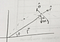# Equations of Motion for a Projectile in Polar Coordinates

Why? There is no why.

Suppose a ball is shot at an angle θ with an initial velocity v 0. What will the motion be like in polar coordinates?

First, let me start with Newton’s 2nd Law in polar coordinates.

Where do those come from? Here is my derivation of the acceleration in polar coordinates. For fun.

Once the ball is in the air (and ignoring air resistance) the only force on the ball is the gravitational force. Yes, this would be -mg in the y-direction, but we don’t have a y-direction. Instead, we have polar coordinates. Maybe this picture will help.Photo: Rhett Allain. Projectile Motion in Polar Coordinates

The r and θ components of the gravitational force will change as:

If I use these forces with Newton’s law in polar coordinates, I get:

Of course the mass cancels — but now I can solve the first equation for (r-double dot) and the second equation for (theta-double dot).

It doesn’t matter that these second derivatives depend on the other stuff — I can still calculate them. Once I have those, I can create a numerical calculation to update the velocities and positions. Suppose I have everything know at time(1), then the stuff at time(2) would be:

Now I’m all set to do a numerical calculation. Well, I still need the initial conditions. I could use this:

But wait! There’s a problem. The calculation for (theta-double dot) has a 1/r term. If r is zero the universe will explode. I can fix this by having the initial r a little bit bigger than zero. Problem solved.

Here is the program in Glowscript.

For the first run, I am going to calculate the x- and y-coordinates in each step and plot x vs y. I know this should look like a parabola.

Boom. That works. Now for a plot of both r and theta as a function of time for a high launch angle.

Double boom.

Originally published at http://rhettallain.com on March 4, 2019.

Physics faculty, science blogger of all things geek. Technical Consultant for CBS MacGyver and MythBusters. Former WIRED blogger.

Physics faculty, science blogger of all things geek. Technical Consultant for CBS MacGyver and MythBusters. Former WIRED blogger.